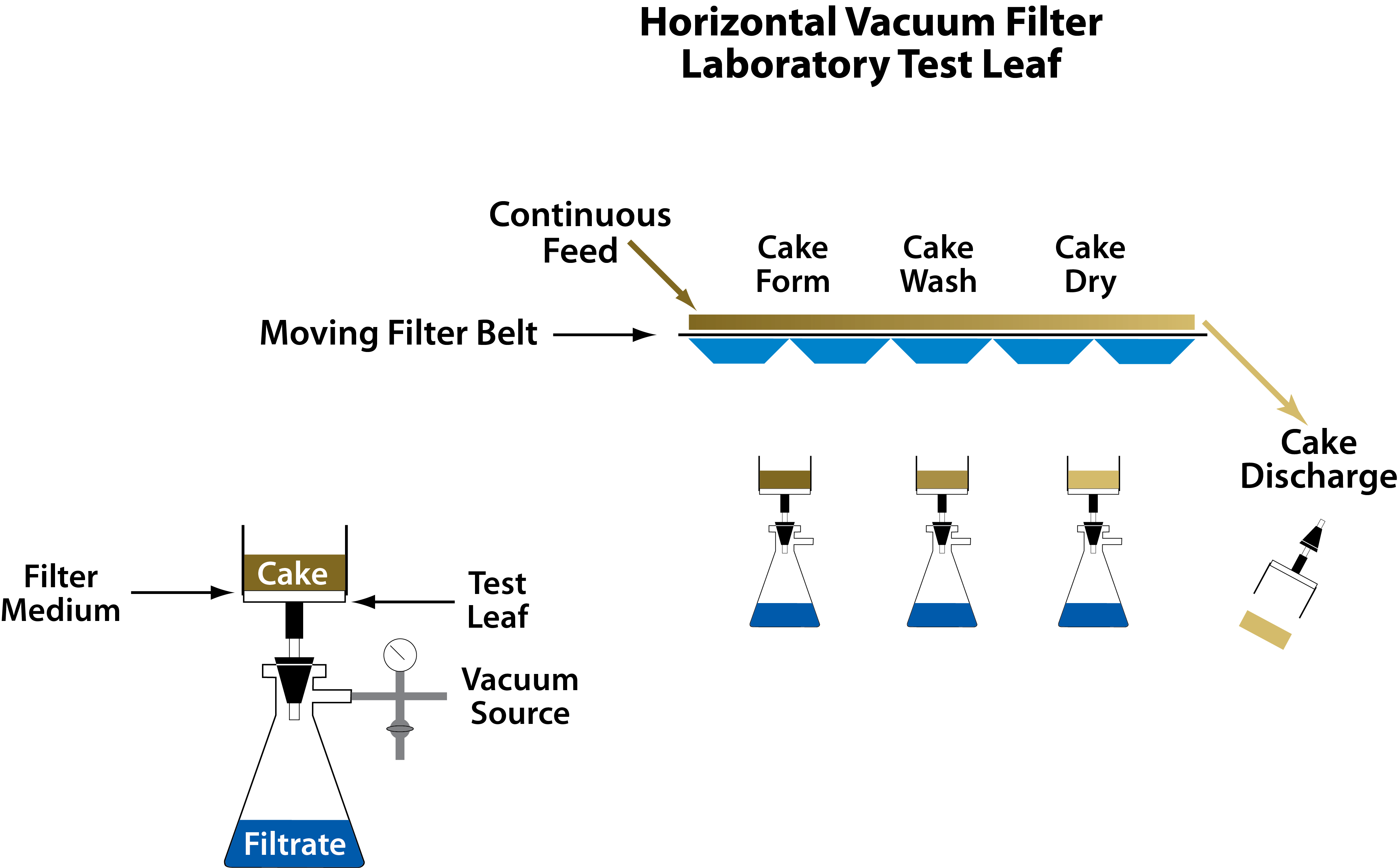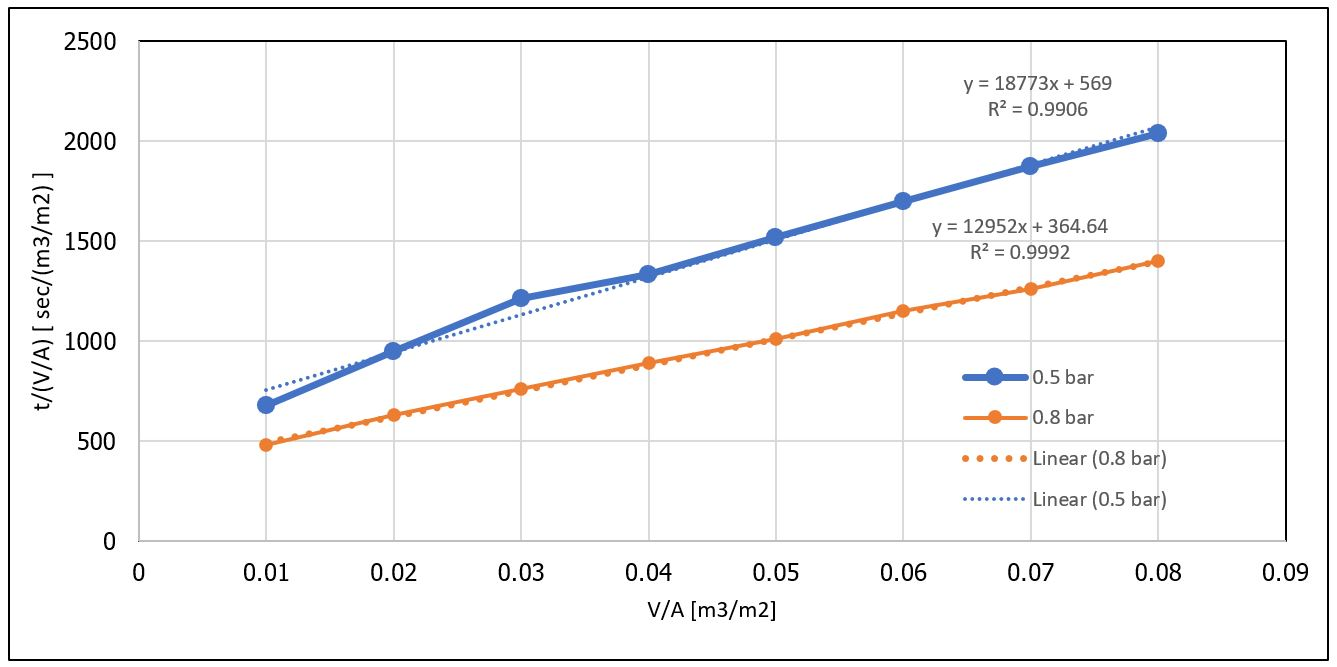# How to Determine Cake Resistance in Filtration Systems

Purpose of this post is to determine cake resistance in filtration systems, as a function of operating pressure of filter. Before we jump into the calculation, let us learn first several basic calculation in filtration system.

As filtration proceeds, a porous cake of solid particles is built up on a porous medium, usually a supported cloth. The flow of liquid is laminar because of the fineness of the pores. Therefore, the following equation represented the phenomena:$Q = \frac{{dV}}{{dt}} = \frac{{A\Delta P}}{{\mu R}}$

The resistance is made up of filter cloth Rf and that of cake Rc which may be assumed proportional to the weight of the cake.$Q = \frac{{dV}}{{dt}} = \frac{{A\Delta P}}{{\mu ({R_f} + {R_C})}} = \frac{{A\Delta P}}{{\mu ({R_f} + \alpha cV/A)}}$

Where:

α = specific resistance of the cake (m/kg)

c = weight of solids per volume of liquid (kg/m3)

μ = viscosity (N sec/m2)

P = pressure difference (N/m2)

A = filtering surface (m2)

V = volume of filtrate (m3)

Q = rate of filtrate accumulation (m3/sec)

Rf and α are constants of the equipment and slurry and must be evaluated from experimental data.

The simplest data to analyze are those obtained from constant pressure or constant rate tests for which the equations will be developed.

At constant pressure, the equation above is integrated as linear form as below:$\frac{t}{{V/A}} = \frac{\mu }{{\Delta P}}{R_f} + \frac{{\mu \alpha c}}{{2\Delta P}}\frac{V}{A}\$

The constants Rf and α are derivable from intercept and slope of the plot t/V against V.

Resistivity of filter cakes depends on the conditions of formation of which the pressure is the major one. At increasing pressures, the particles or aggregates may be distorted and brought closer together. Equation below show relationship between specific resistivity of cake and pressure differences.$\alpha = {\alpha _0}{(\Delta P)^n}\$

## Example

In this example, we want to determine filter cloth resistance and cake resistance as a function of pressure from laboratory filtration test.Filtration tests were performed on calcium carbonate slurry with the following properties:

Weight of solids per volume of liquid (c) = 135 kg solid/m3 solid

Dynamic viscosity (μ) = 0.001 N sec/m2

Area of the filter leaf was 500 cm2.

Data were taken of the volume of the filtrate (L) against time (sec) at pressures of 0.5 and 0.8 bar. The results will be analyzed for the filtration parameters:

 Vol of Filtrate (L) V/A (m3/m2) 0.5 bar 0.8 bar t t/(V/A) t t/(V/A) 0.5 0.01 6.8 680 4.8 480 1 0.02 19.0 950 12.6 630 1.5 0.03 36.4 1213 22.8 760 2 0.04 53.4 1335 35.6 890 2.5 0.05 76.0 1520 50.5 1010 3 0.06 102.0 1700 69 1150 3.5 0.07 131.2 1874 88.2 1260 4 0.08 163.0 2038 112 1400 4.5 0.09 5 0.1

The first step is to create a figure as a function of V/A and t/(V/A).Based on intercept, we can obtain resistance of filter cloth (Rf ) and based on slope we can get specific resistivity of cake (α).

 Pressure (bar) Pressure (Pa) Slope Intercept Rf (Resistance of filter cloth) (m-1) α (specific resistance of cake) (m/kg) 0.5 50000 18773 569 2.8.E+10 1.4.E+10 0.8 80000 12952 365 2.9.E+10 1.5.E+10

Therefore, resistance of filter cloth is around 3 x 1010 per meter.

As for specific resistivity of cake as function of pressure:

α = kPn

where n = ln(α12)/ln(P1/P2) = 0.21

k = α/Pn

k = 1.61 x 1010

Therefore, α = 1.61 x 1010 P0.21